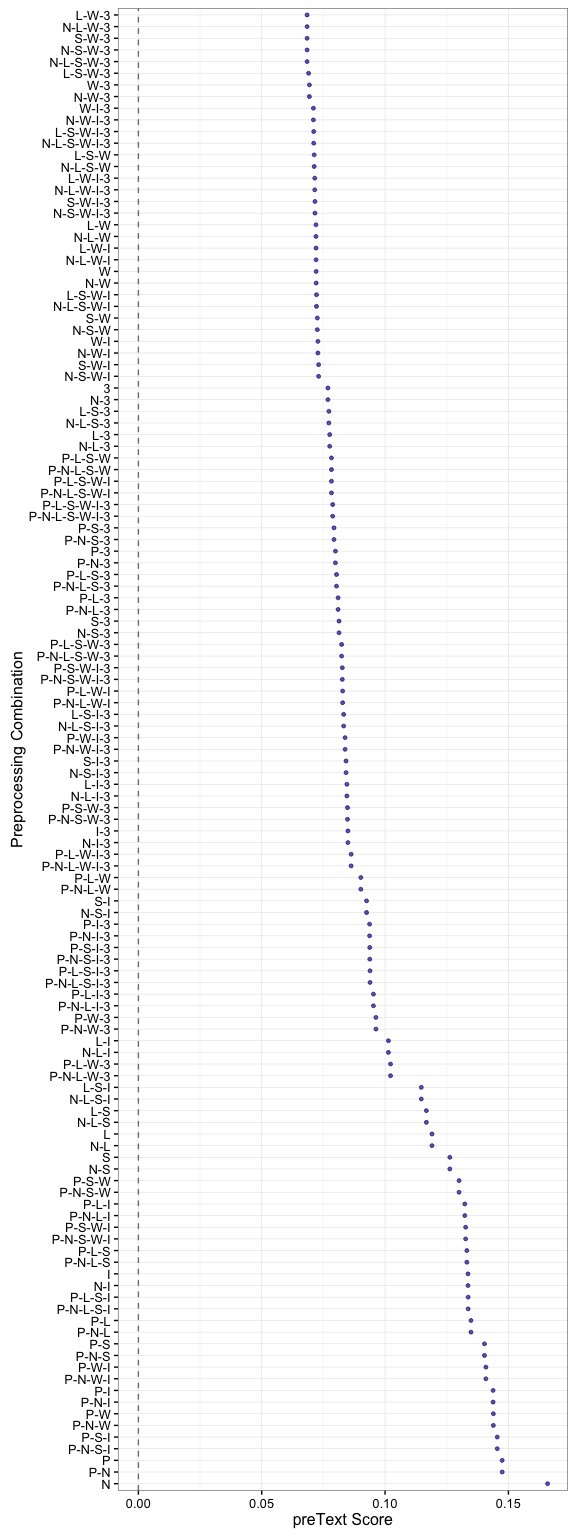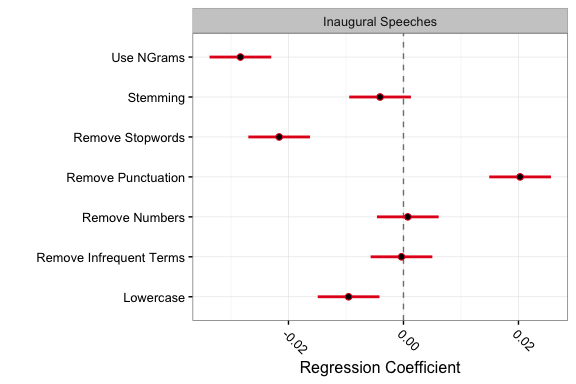# Getting Started With preText

#### 2016-10-06

This vignette is designed to introduce you to the preText R package. This package is built on top of the quanteda R package for text processing and can take as input a quanteda::corpus object, or a character vector (with one string per document). The main functions will preprocess the input text 64-128 different ways, and then allow the user to assess the consequences of the different preprocessing steps based on how unusual the resulting document–term matrix (DTM) is using the preText procedure.

Our paper detailing the preText procedure can be found at the link below:

• Matthew J. Denny, and Arthur Spirling (2016). “Assessing the Consequences of Text Preprocessing Decisions”. [ssrn.com/abstract=2849145]

## Installation

The release version of the package can be installed from CRAN as follows:

install.packages("preText")

If you want to get the latest version from GitHub, start by checking out the Requirements for using C++ code with R section in the following tutorial: Using C++ and R code Together with Rcpp. You will likely need to install either Xcode or Rtools depending on whether you are using a Mac or Windows machine before you can install the preText package via GitHub, since it makes use of C++ code.

install.packages("devtools")

Now we can install from GitHub using the following line:

devtools::install_github("matthewjdenny/preText")

Once the GERGM package is installed, you may access its functionality as you would any other package by calling:

library(preText)

If all went well, check out the vignette("getting_started_with_preText") which will pull up this vignette!

## Basic Usage

We begin by loading the package and some example data from the quanteda R package. In this example, we will make use of 57 U.S. presidential inaugural speeches. As a general rule, you will want to limit the number of documents used with preText to several hundred in most cases, in order to avoid extremely long run times and/or high memory requirements. To make this example run more quickly, we are only going to use 10 documents.

library(preText)
library(quanteda)

# load in U.S. presidential inaugural speeches from Quanteda example data.
corp <- corpus(inaugTexts)
# use first 10 documents for example
documents <- texts(corp)[1:10]
# take a look at the document names
print(names(documents))
##   "1789-Washington" "1793-Washington" "1797-Adams"
##  "1825-Adams"

Having loaded in some data, we can now make use of the factorial_preprocessing() function, which will preprocess the data 64 or 128 different ways (depending on whether n-grams are included). In this example, we are going to preprocess the documents all 128 different ways. This should take between 5 and 10 minutes on most modern laptops. Longer documents and larger numbers of documents will significantly increase run time and memory usage. It is highly inadvisable to use more than 500-1,000 under any circumstances and in the case where the user wishes to preprocess more than a few hundred documents, they may want to explore the parallel option. This can significantly speed up preprocessing, but will require significantly more RAM on the computer being used. Here, we have selected the use_ngrams = TRUE option, and set the document proportion threshold at which to remove infrequent terms at 0.2. This means that terms which appear in less than 20 percent (2/10) documents will be removed. The default value is 0.01 (or 1/100 documents), but for this small corpus, we increase the value. In order prevent spamming this vignette with output, we have elected to set the verbose option to FALSE. In practice, it is better to keep verbose = TRUE to make it easier to evaluate the progress of preprocessing.

preprocessed_documents <- factorial_preprocessing(
documents,
use_ngrams = TRUE,
infrequent_term_threshold = 0.2,
verbose = FALSE)
## Preprocessing 10 documents 128 different ways...

## This function will output a list object with three fields. The first of these is $choices, a data.frame containing indicators for each of the preprocessing steps used. The second is $dfm_list, which is a list with 64 or 128 entries, each of which contains a quanteda::dfm object preprocessed according to the specification in the corresponding row in choices. Each DFM in this list will be labeled to match the row names in choices, but you can also access these labels from the $labels field. We can look at the first few rows of choices below: names(preprocessed_documents) ##  "choices" "dfm_list" "labels" head(preprocessed_documents$choices)
##               removePunctuation removeNumbers lowercase stem
## P-N-L-S-W-I-3              TRUE          TRUE      TRUE TRUE
## N-L-S-W-I-3               FALSE          TRUE      TRUE TRUE
## P-L-S-W-I-3                TRUE         FALSE      TRUE TRUE
## L-S-W-I-3                 FALSE         FALSE      TRUE TRUE
## P-N-S-W-I-3                TRUE          TRUE     FALSE TRUE
## N-S-W-I-3                 FALSE          TRUE     FALSE TRUE
##               removeStopwords infrequent_terms use_ngrams
## P-N-L-S-W-I-3            TRUE             TRUE       TRUE
## N-L-S-W-I-3              TRUE             TRUE       TRUE
## P-L-S-W-I-3              TRUE             TRUE       TRUE
## L-S-W-I-3                TRUE             TRUE       TRUE
## P-N-S-W-I-3              TRUE             TRUE       TRUE
## N-S-W-I-3                TRUE             TRUE       TRUE

Now that we have our preprocessed documents, we can perform the preText procedure on the factorial preprocessed corpus using the preText() function. It will be useful now to give a name to our data set using the dataset_name argument, as this will show up in some of the plots we generate with the output. The standard number of pairs to compare is 50 for reasonably sized corpora, but because we are only using 10 documents, the maximum number of pairwise document distances is only (10)*(10 - 1)/2 = 45, so we select 20 pairwise comparisons for purposes of illustration. This function will usually not take as long to run as the factorial_preprocessing() function, but parallelization is also available for this function if a speedup is desired. It is suggested that the user select verbose = TRUE in practice, but we set it to FALSE here to avoid cluttering this vignette. This function should run in 10-30 seconds for this small corpora, and in several hours to a day for most moderately sized corpora.

preText_results <- preText(
preprocessed_documents,
dataset_name = "Inaugural Speeches",
distance_method = "cosine",
num_comparisons = 20,
verbose = FALSE)
## Generating document distances...
## Generating preText Scores...
## Generating regression results..
## Regression results (negative coefficients imply less risk):
##                  Variable Coefficient    SE
## 1               Intercept       0.117 0.004
## 2      Remove Punctuation       0.020 0.003
## 3          Remove Numbers       0.001 0.003
## 4               Lowercase      -0.010 0.003
## 5                Stemming      -0.004 0.003
## 6        Remove Stopwords      -0.022 0.003
## 7 Remove Infrequent Terms       0.000 0.003
## 8              Use NGrams      -0.028 0.003
## Complete in: 12.676 seconds...

The preText() function returns a list of result with four fields:

• $preText_scores: A data.frame containing preText scores and preprocessing step labels for each preprocessing step as columns. Note that there is no preText score for the case of no prepprocessing steps. • $ranked_preText_scores: A data.frame that is identical to $preText_scores except that it is ordered by the magnitude of the preText score • $choices: A data.frame containing binary indicators of which preprocessing steps were applied to factorial preprocessed DFM.
• \$regression_results: A data.frame containing regression results where indicators for each preprocessing decision are regressed on the preText score for that specification.

We can now feed these results to two functions that will help us make better sense of them. preText_score_plot() creates a dot plot of scores for each preprocessing specification:

preText_score_plot(preText_results)Here, the least risky specifications have the lowest preText score and are displayed at the top of the plot. We can also see the conditional effects of each preprocessing step on the mean preText score for each specification that included that step. Here again, a negative coefficient indicates that a step tends to reduce the unusualness of the results, while a positive coefficient indicates that applying the step is likely to produce more unusual results for that corpus.

regression_coefficient_plot(preText_results,
remove_intercept = TRUE)In this particular toy example, we see that including n-grams and removing stop words tends to produce more normal results, while removing punctuation tends to produce more unusual results. However, we should not put too much stock in these results due to the small number of documents used to generate them.

The preText package provides a number of additional functions for examining the effects of preprocessing decisions on resulting DFMs. Please see the README on the package GitHub page for more details on these additional functions.

## Replication of Results for UK Manifestos Data

The example provided above uses a toy dataset, mostly to reduce the runtime for the analysis to under 20 minutes on most computers. For those who would like to explore a full example, we include the UK Manifestos dataset described in the paper with our package. It can be accessed using the data("UK_Manifestos") command once the package is loaded. Below, we provide a full working example which will replicated results from the paper. The code has not been run in this vignette to save time, but you may give it a try on your own computer. It should run in less than 24 hours on most computers.

# load the package
library(preText)
data("UK_Manifestos")
# preprocess data
preprocessed_documents <- factorial_preprocessing(
UK_Manifestos,
use_ngrams = TRUE,
infrequent_term_threshold = 0.02,
verbose = TRUE)
# run preText
preText_results <- preText(
preprocessed_documents,
dataset_name = "Inaugural Speeches",
distance_method = "cosine",
num_comparisons = 100,
verbose = TRUE)
# generate preText score plot
preText_score_plot(preText_results)
# generate regression results
regression_coefficient_plot(preText_results,
remove_intercept = TRUE)